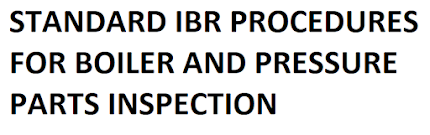### How do you calculate Steam turbine heat rate and efficiency???

How do you calculate the Turbine efficiency?

Turbine efficiency is the ratio of heat out put to the heat input

Here, heat input is heat content in inlet steam and out put is power generation.

Actually, total  power generation =Power generated on generator terminal / (Gear box efficiency X Alternator efficiency).

What is Turbine heat rate?

Turbine heat rate is the ratio of total heat required to generate unit power.

Turbine efficiency = 860 X 100 / Turbine heat rate

Thermal power plant turbine heat rate

= Steam flow (Main steam enthalpy-Feed water enthalpy) / Power generation

Co-generation Turbine heat rate:

=((Steam Flow x Steam Enthalpy +Makeup Water flow x Its Enthalpy+ RC Flow x RC Enthalpy)-(Process-1 steam flow x its Enthalpy + Process-2 steam flow x Its Enthalpy+ FW Flow x FW Enthalpy)) /Power Generation

Co-generation Turbine heat rate is also calculated as;

((Steam Flow x Steam Enthalpy)-(1St EXT Flow x Its Enthalpy + 2nd Ext flow x its Enthalpy + 3rd Ext flow x Its Enthalpy+ Exhaust Steam flow x its Enthalpy)) /Power Generation

Calculations:

A thermal power plant is generating 55 MW power at specific steam consumption 3.7 MT/MW and at pressure and temperature 110 kg/cm2 and 540 deg C respectively.Calculate the Turbine heat rate and  efficiency if its feed water inlet temperature at economizer is 215 deg C

Turbine inlet steam flow = 55 X 3.7 = 203.5 TPH

Enthalpy of inlet steam at rated pressure and temperatures = 821 kcal/kg

Enthalpy of feed water = 217 kcal/kg

Thermal power plant turbine heat rate= Steam flow (Main steam enthalpy-Feed water enthalpy) / Power generation

THR = 203.5 X (821-217) / 55

THR = 2234.8 kcal/kwh

Turbine efficiency = 860 X 100 / 2234.8

Turbine efficiency = 38.48%

A back pressure turbine is generating 7.5 MW load at specific steam consumption 7.5 MT/MW, calculate the turbine heat rate and efficiency if inlet steam pressure and temperatures are 64 kg/cm2 and 485 deg C and exhaust steam parameters are 4 kg/cm2 and 165 deg C

Inlet steam flow = 7.5 X 7.5 = 56.25 TPH

Enthalpy of inlet steam= 810 kcal/kg

Enthalpy of exhaust steam = 665 kcal/kg

Turbine heat rate = (Inlet steam flow X Enthalpy-Exhaust steam flow X Enthalpy) / Power generation

Turbine heat rate =(56.25 X 810-56.25 X 665) / 7.5

Turbine heat rate = 1087.5 kcal/kw

Turbine efficiency = 860 X 100/ Heat rate

= 860 X 100/1087.5

= 79%

The operation details of a 22 MW condensing cum extraction turbines are as below, calculate its heat rate & efficiency

 SL No Particular UOM Value 1 Turbine inlet steam pressure Kg/cm2 88 2 Turbine inlet steam temperature Deg C 515 3 Turbine inlet steam flow TPH 120 4 Process steam flow TPH 65 5 Process steam pressure Kg/cm2 2.0 6 Process steam temperature Deg C 140 7 Exhaust steam pressure Kg/cm2 -0.88 7a Exhaust steam flow TPH 55 8 Process return condensate flow TPH 58 9 Process condensate temperature Deg C 115 10 DM Make up water flow TPH 9 11 DM Make up water temperature Deg C 27 12 Feed water flow TPH 121 13 Feed water temperature Deg C 145

Solution:

Enthalpy of turbine inlet steam = 818 kcal/kg

Enthalpy of process steam =655 kcal/kg

Enthalpy of feed water= 147 kcal/kg

Enthalpy of return condensate=116 kcal/kg

Enthalpy of DM water = 25 kcal/kg

Enthalpy of exhaust steam in( liquid form) = 52 kcal/kg

Co-generation Turbine heat rate:

=((Steam Flow x Steam Enthalpy +Makeup Water flow x Its Enthalpy+ RC Flow x RC Enthalpy)-(Process-steam flow x its Enthalpy + FW Flow x FW Enthalpy)) /Power Generation

=((120 X 818 + 9 X 25 + 58 X 115)-(65 X 655 + 121 X 147)) / 22

=2031.5 kcal/kg

Turbine efficiency = 860 X 100 / 2031.5

= 42.33%

Same can be calculated by another formula

THR=((Steam Flow x Steam Enthalpy)-(EXT Flow x Its Enthalpy ++ Exhaust Steam flow x its Enthalpy)) /Power Generation

THR =(120 X 818 -65 X 655 - 55 X 52) / 22

THR =2396.5 kcal/kg

Turbine efficiency = 860 X 100 / 2396.5

= 35.88%